Chapter 2 Class 10 Polynomials
Serial order wise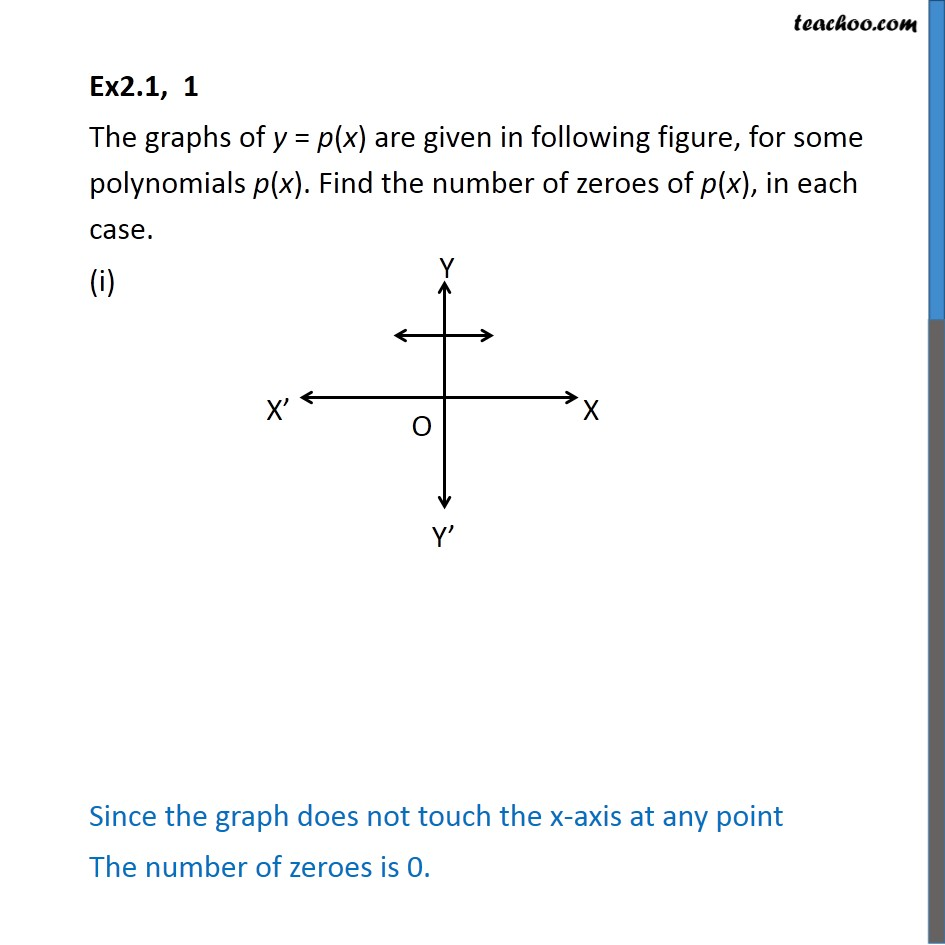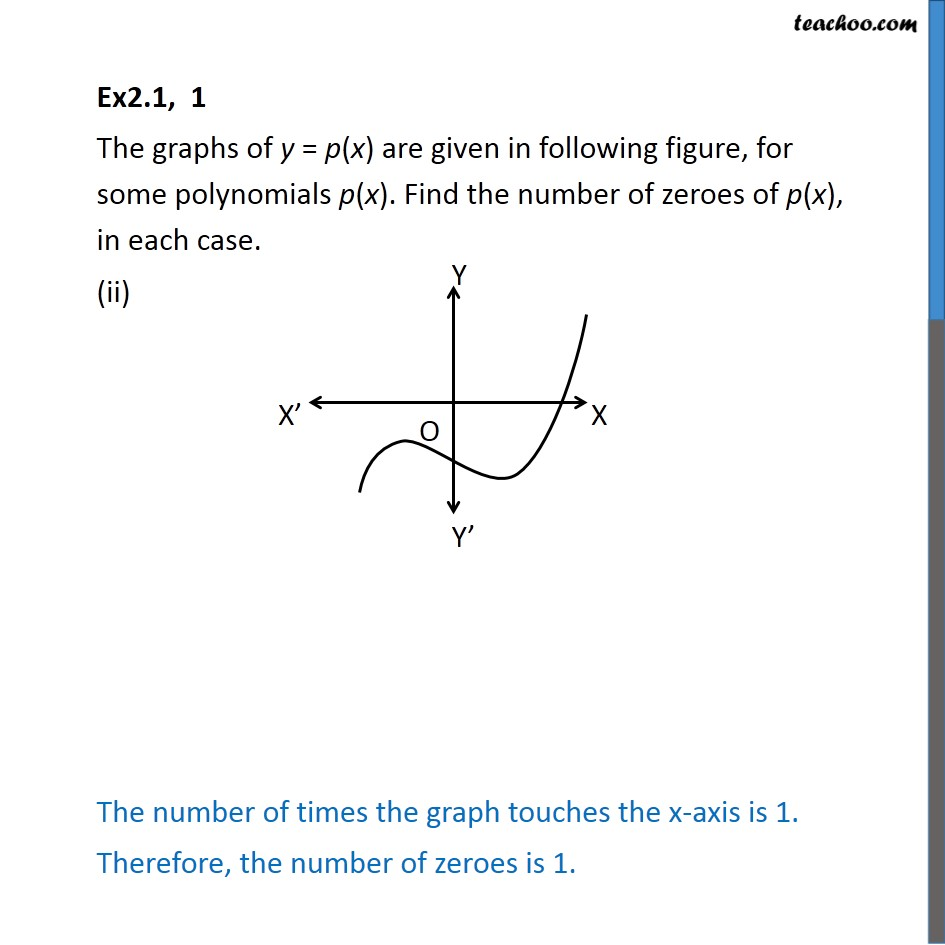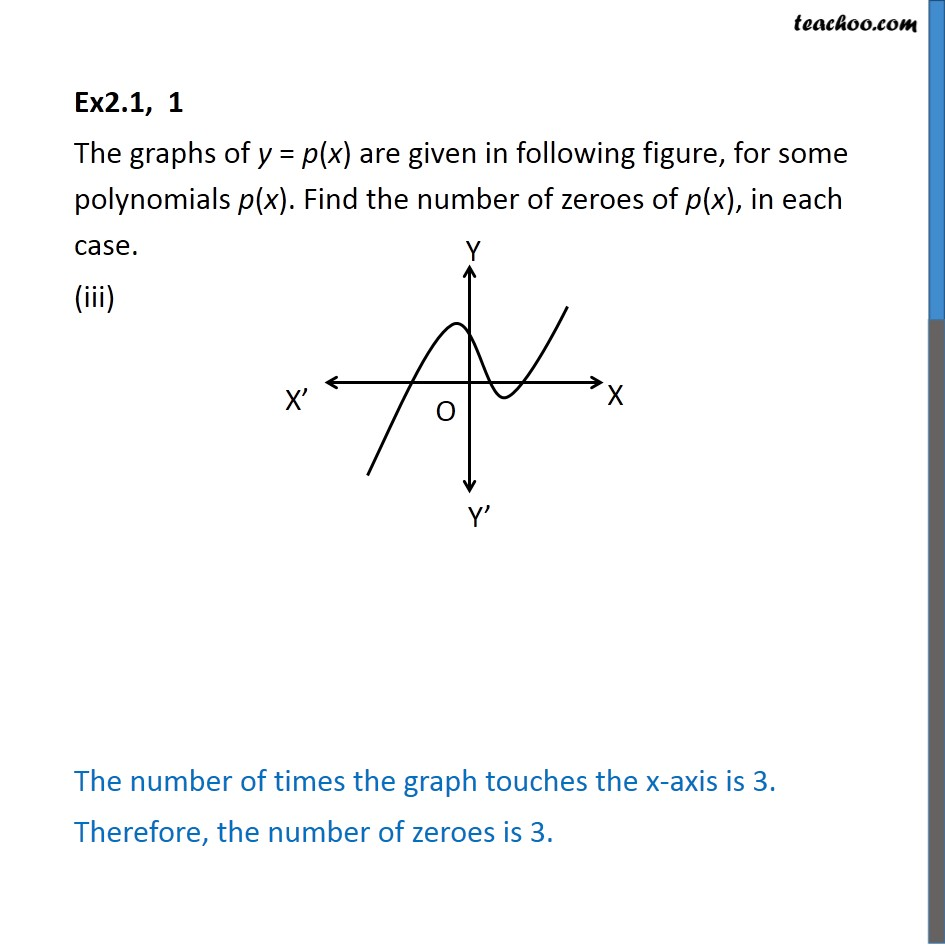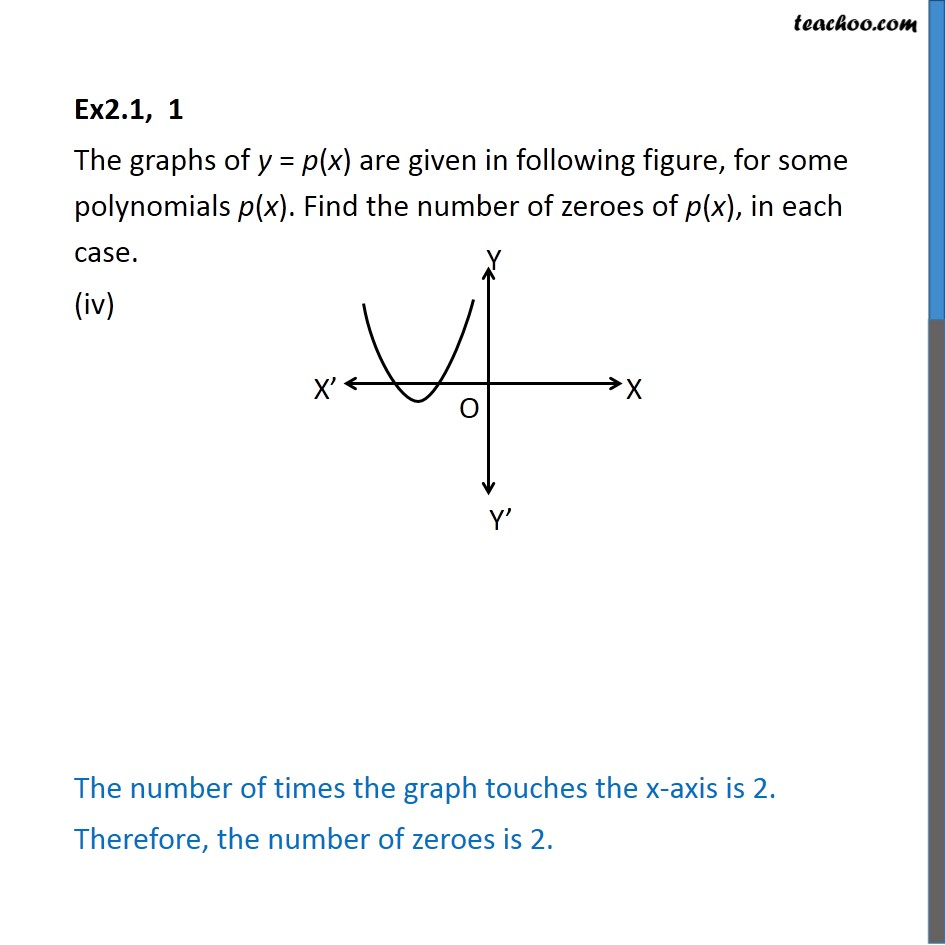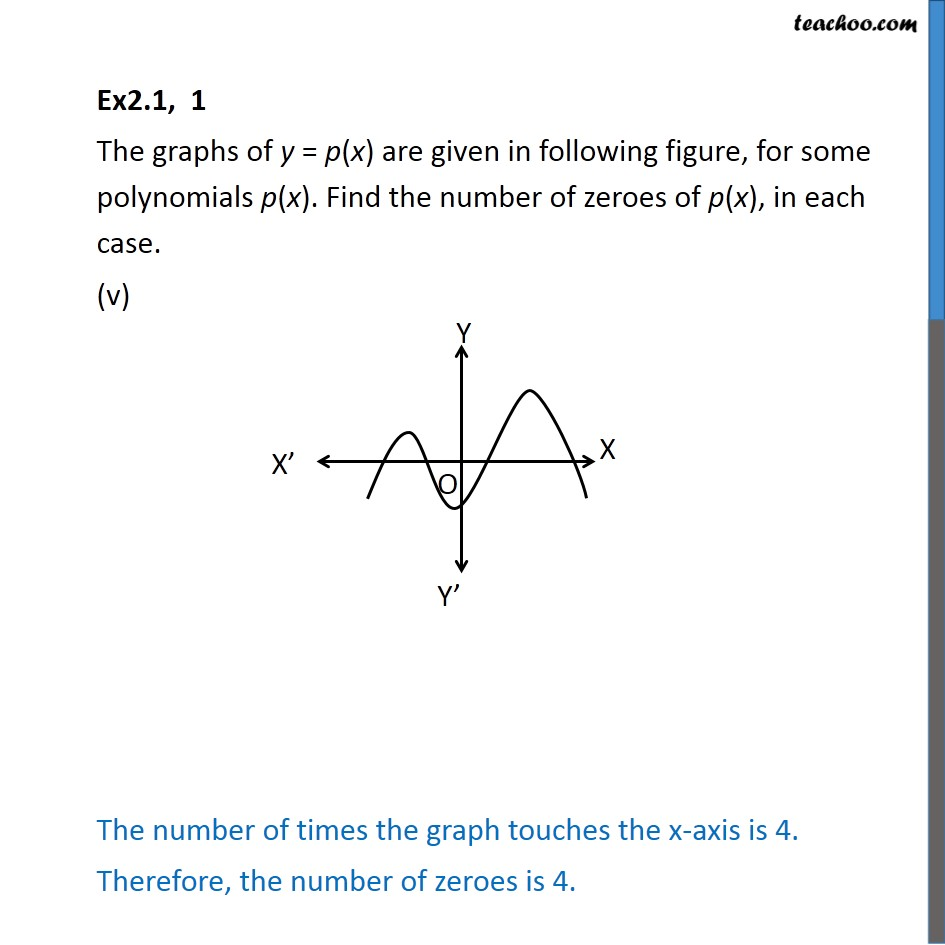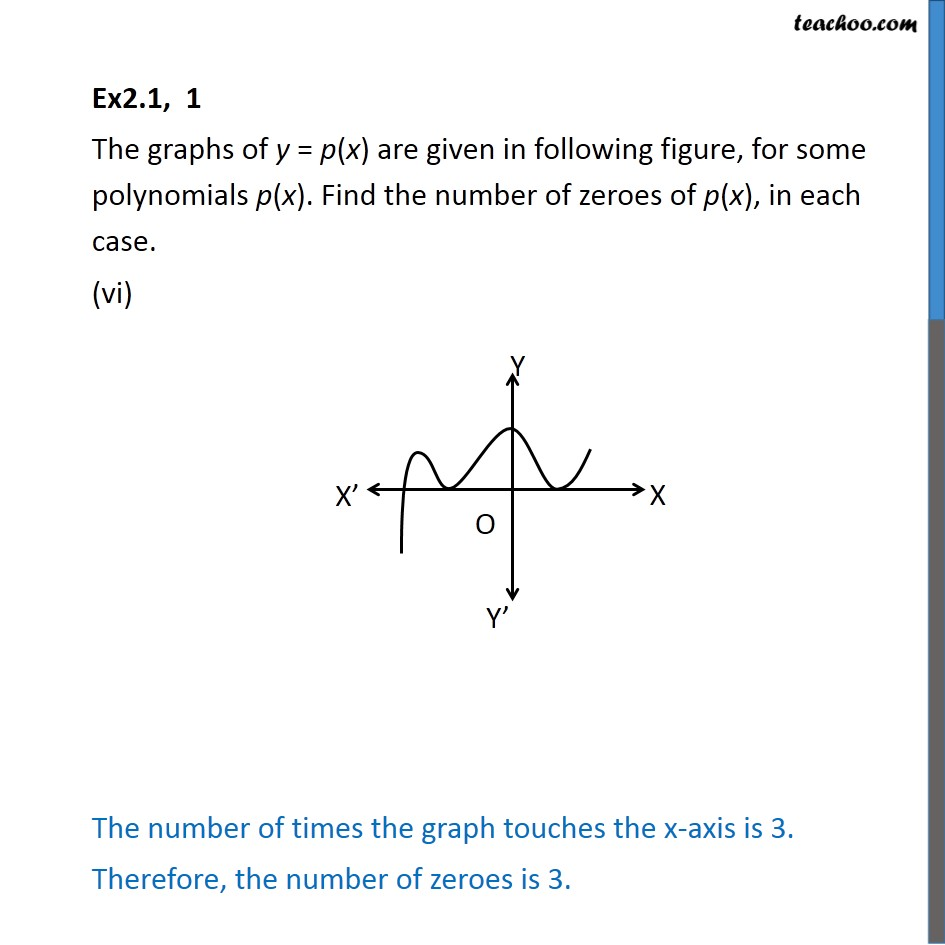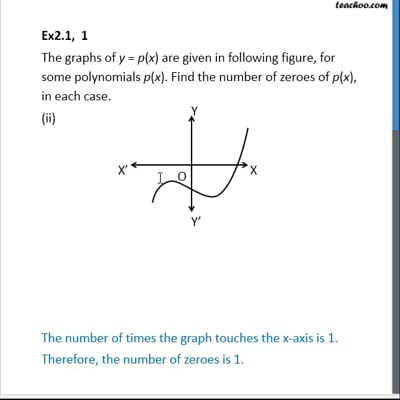This video is only available for Teachoo black users

Solve all your doubts with Teachoo Black (new monthly pack available now!)

### Transcript

Ex2.1, 1 The graphs of y = p(x) are given in following figure, for some polynomials p(x). Find the number of zeroes of p(x), in each case. (i) Since the graph does not touch the x-axis at any point The number of zeroes is 0. Ex2.1, 1 The graphs of y = p(x) are given in following figure, for some polynomials p(x). Find the number of zeroes of p(x), in each case. (ii) The number of times the graph touches the x-axis is 1. Therefore, the number of zeroes is 1. Ex2.1, 1 The graphs of y = p(x) are given in following figure, for some polynomials p(x). Find the number of zeroes of p(x), in each case. (iii) The number of times the graph touches the x-axis is 3. Therefore, the number of zeroes is 3. Ex2.1, 1 The graphs of y = p(x) are given in following figure, for some polynomials p(x). Find the number of zeroes of p(x), in each case. (iv) The number of times the graph touches the x-axis is 2. Therefore, the number of zeroes is 2. Ex2.1, 1 The graphs of y = p(x) are given in following figure, for some polynomials p(x). Find the number of zeroes of p(x), in each case. (v) The number of times the graph touches the x-axis is 4. Therefore, the number of zeroes is 4. Ex2.1, 1 The graphs of y = p(x) are given in following figure, for some polynomials p(x). Find the number of zeroes of p(x), in each case. (vi) The number of times the graph touches the x-axis is 3. Therefore, the number of zeroes is 3.